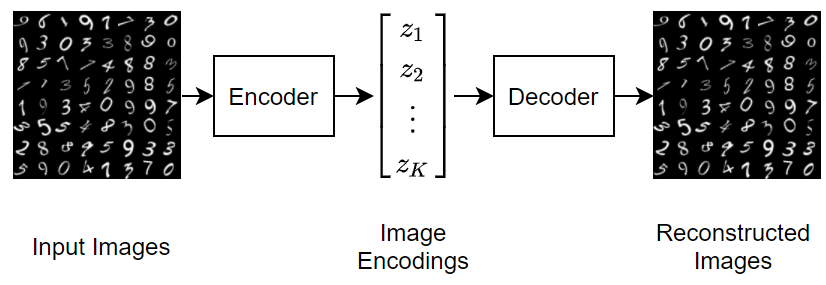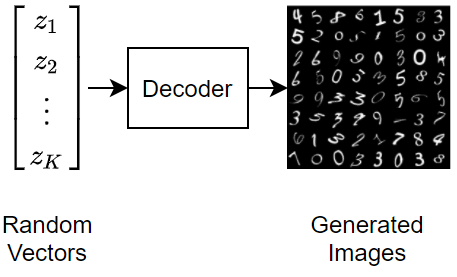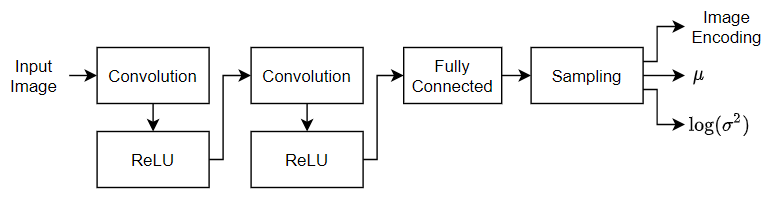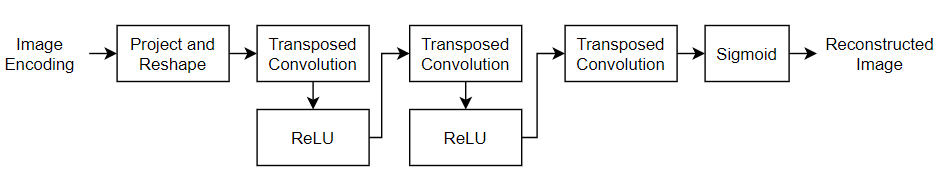# Train Variational Autoencoder (VAE) to Generate Images

This example shows how to create a variational autoencoder (VAE) in MATLAB to generate digit images. The VAE generates hand-drawn digits in the style of the MNIST data set.

VAEs differ from regular autoencoders in that they do not use the encoding-decoding process to reconstruct an input. Instead, they impose a probability distribution on the latent space, and learn the distribution so that the distribution of outputs from the decoder matches that of the observed data. Then, they sample from this distribution to generate new data.

In this example, you construct a VAE network, train it on the MNIST data set, and generate new images that closely resemble those in the data set.

Download the MNIST files from http://yann.lecun.com/exdb/mnist/ and load the MNIST data set into the workspace . Extract and place the files in the working directory, then call the `processImagesMNIST` and `processLabelsMNIST` helper functions attached to this example to load the data from the files into MATLAB arrays.

Because the VAE compares the reconstructed digits against the inputs and not against the categorical labels, you do not need to use the training labels in the MNIST data set.

```trainImagesFile = 'train-images.idx3-ubyte'; testImagesFile = 't10k-images.idx3-ubyte'; testLabelsFile = 't10k-labels.idx1-ubyte'; XTrain = processImagesMNIST(trainImagesFile);```
```Read MNIST image data... Number of images in the dataset: 60000 ... ```
```numTrainImages = size(XTrain,4); XTest = processImagesMNIST(testImagesFile);```
```Read MNIST image data... Number of images in the dataset: 10000 ... ```
`YTest = processLabelsMNIST(testLabelsFile);`
```Read MNIST label data... Number of labels in the dataset: 10000 ... ```

### Construct Network

Autoencoders have two parts: the encoder and the decoder. The encoder takes an image input and outputs a compressed representation (the encoding), which is a vector of size `latent_dim`, equal to 20 in this example. The decoder takes the compressed representation, decodes it, and recreates the original image.

To make calculations more numerically stable, increase the range of possible values from [0,1] to [-inf, 0] by making the network learn from the logarithm of the variances. Define two vectors of size `latent_dim`: one for the means $\mu$ and one for the logarithm of the variances $\mathrm{log}\left({\sigma }^{2}\right)$. Then use these two vectors to create the distribution to sample from.

Use 2-D convolutions followed by a fully connected layer to downsample from the 28-by-28-by-1 MNIST image to the encoding in the latent space. Then, use transposed 2-D convolutions to scale up the 1-by-1-by-20 encoding back into a 28-by-28-by-1 image.

```latentDim = 20; imageSize = [28 28 1]; encoderLG = layerGraph([ imageInputLayer(imageSize,'Name','input_encoder','Normalization','none') convolution2dLayer(3, 32, 'Padding','same', 'Stride', 2, 'Name', 'conv1') reluLayer('Name','relu1') convolution2dLayer(3, 64, 'Padding','same', 'Stride', 2, 'Name', 'conv2') reluLayer('Name','relu2') fullyConnectedLayer(2 * latentDim, 'Name', 'fc_encoder') ]); decoderLG = layerGraph([ imageInputLayer([1 1 latentDim],'Name','i','Normalization','none') transposedConv2dLayer(7, 64, 'Cropping', 'same', 'Stride', 7, 'Name', 'transpose1') reluLayer('Name','relu1') transposedConv2dLayer(3, 64, 'Cropping', 'same', 'Stride', 2, 'Name', 'transpose2') reluLayer('Name','relu2') transposedConv2dLayer(3, 32, 'Cropping', 'same', 'Stride', 2, 'Name', 'transpose3') reluLayer('Name','relu3') transposedConv2dLayer(3, 1, 'Cropping', 'same', 'Name', 'transpose4') ]);```

To train both networks with a custom training loop and enable automatic differentiation, convert the layer graphs to `dlnetwork` objects.

```encoderNet = dlnetwork(encoderLG); decoderNet = dlnetwork(decoderLG);```

The helper function modelGradients takes in the encoder and decoder `dlnetwork` objects and a mini-batch of input data `X`, and returns the gradients of the loss with respect to the learnable parameters in the networks. This helper function is defined at the end of this example.

The function performs this process in two steps: sampling and loss. The sampling step samples the mean and the variance vectors to create the final encoding to be passed to the decoder network. However, because backpropagation through a random sampling operation is not possible, you must use the reparameterization trick. This trick moves the random sampling operation to an auxiliary variable $\epsilon$, which is then shifted by the mean ${\mu }_{\mathit{i}}$ and scaled by the standard deviation ${\sigma }_{\mathit{i}}$. The idea is that sampling from $\mathit{N}\left({\mu }_{\mathit{i}},{\sigma }_{\mathit{i}}^{2}\right)$ is the same as sampling from ${\mu }_{\mathit{i}}+\epsilon \cdot {\sigma }_{\mathit{i}}$, where $\epsilon \sim \mathit{N}\left(0,1\right)$. The following figure depicts this idea graphically.The loss step passes the encoding generated by the sampling step through the decoder network, and determines the loss, which is then used to compute the gradients. The loss in VAEs, also called the evidence lower bound (ELBO) loss, is defined as a sum of two separate loss terms:

$\mathrm{ELBO}\text{\hspace{0.17em}}\mathrm{loss}=\mathrm{reconstruction}\text{\hspace{0.17em}}\mathrm{loss}+\mathrm{KL}\text{\hspace{0.17em}}\mathrm{loss}$.

The reconstruction loss measures how close the decoder output is to the original input by using the mean-squared error (MSE):

$\mathrm{reconstruction}\text{\hspace{0.17em}}\mathrm{loss}=\mathrm{MSE}\left(\mathrm{decoder}\text{\hspace{0.17em}}\mathrm{output},\mathrm{original}\text{\hspace{0.17em}}\mathrm{image}\right)$.

The KL loss, or Kullback–Leibler divergence, measures the difference between two probability distributions. Minimizing the KL loss in this case means ensuring that the learned means and variances are as close as possible to those of the target (normal) distribution. For a latent dimension of size $\mathit{n}$, the KL loss is obtained as

$\mathrm{KL}\text{\hspace{0.17em}}\mathrm{loss}=-0.5\cdot \sum _{\mathit{i}=1}^{\mathit{n}}\left(1+\mathrm{log}\left({\sigma }_{\mathit{i}}\right)-{\mu }_{\mathit{i}}^{2}-{\sigma }_{\mathit{i}}^{2}\right)$.

The practical effect of including a KL loss term is to pack the clusters learned due to the reconstruction loss tightly around the center of the latent space, forming a continuous space to sample from.

### Specify Training Options

Train on a GPU (requires Parallel Computing Toolbox™). If you do not have a GPU, set the `executionEnvironment` to `"cpu"`.

`executionEnvironment = "auto";`

Set the training options for the network. When using the Adam optimizer, you need to initialize for each network the trailing average gradient and the trailing average gradient-square decay rates with empty arrays`.`

```numEpochs = 50; miniBatchSize = 512; lr = 1e-3; numIterations = floor(numTrainImages/miniBatchSize); iteration = 0; avgGradientsEncoder = []; avgGradientsSquaredEncoder = []; avgGradientsDecoder = []; avgGradientsSquaredDecoder = [];```

### Train Model

Train the model using a custom training loop.

For each iteration in an epoch:

• Obtain the next mini-batch from the training set.

• Convert the mini-batch to a `dlarray` object, making sure to specify the dimension labels `'SSCB'` (spatial, spatial, channel, batch).

• For GPU training, convert the `dlarray` to a `gpuArray` object.

• Evaluate the model gradients using the `dlfeval `and` modelGradients `functions.

• Update the network learnables and the average gradients for both networks, using the `adamupdate` function.

At the end of each epoch, pass the test set images through the autoencoder, and display the loss and the training time for that epoch.

```for epoch = 1:numEpochs tic; for i = 1:numIterations iteration = iteration + 1; idx = (i-1)*miniBatchSize+1:i*miniBatchSize; XBatch = XTrain(:,:,:,idx); XBatch = dlarray(single(XBatch), 'SSCB'); if (executionEnvironment == "auto" && canUseGPU) || executionEnvironment == "gpu" XBatch = gpuArray(XBatch); end [infGrad, genGrad] = dlfeval(... @modelGradients, encoderNet, decoderNet, XBatch); [decoderNet.Learnables, avgGradientsDecoder, avgGradientsSquaredDecoder] = ... adamupdate(decoderNet.Learnables, ... genGrad, avgGradientsDecoder, avgGradientsSquaredDecoder, iteration, lr); [encoderNet.Learnables, avgGradientsEncoder, avgGradientsSquaredEncoder] = ... adamupdate(encoderNet.Learnables, ... infGrad, avgGradientsEncoder, avgGradientsSquaredEncoder, iteration, lr); end elapsedTime = toc; [z, zMean, zLogvar] = sampling(encoderNet, XTest); xPred = sigmoid(forward(decoderNet, z)); elbo = ELBOloss(XTest, xPred, zMean, zLogvar); disp("Epoch : "+epoch+" Test ELBO loss = "+gather(extractdata(elbo))+... ". Time taken for epoch = "+ elapsedTime + "s") end```
```Epoch : 1 Test ELBO loss = 27.0561. Time taken for epoch = 33.0037s Epoch : 2 Test ELBO loss = 24.414. Time taken for epoch = 32.4167s Epoch : 3 Test ELBO loss = 23.0166. Time taken for epoch = 32.3244s Epoch : 4 Test ELBO loss = 20.9078. Time taken for epoch = 32.1268s Epoch : 5 Test ELBO loss = 20.6519. Time taken for epoch = 32.3451s Epoch : 6 Test ELBO loss = 20.3201. Time taken for epoch = 32.4371s Epoch : 7 Test ELBO loss = 19.9266. Time taken for epoch = 32.4551s Epoch : 8 Test ELBO loss = 19.8448. Time taken for epoch = 32.9919s Epoch : 9 Test ELBO loss = 19.7485. Time taken for epoch = 33.1783s Epoch : 10 Test ELBO loss = 19.6295. Time taken for epoch = 33.1623s Epoch : 11 Test ELBO loss = 19.539. Time taken for epoch = 32.4781s Epoch : 12 Test ELBO loss = 19.4682. Time taken for epoch = 32.5094s Epoch : 13 Test ELBO loss = 19.3577. Time taken for epoch = 32.5996s Epoch : 14 Test ELBO loss = 19.3247. Time taken for epoch = 32.6447s Epoch : 15 Test ELBO loss = 19.3043. Time taken for epoch = 32.2494s Epoch : 16 Test ELBO loss = 19.2948. Time taken for epoch = 32.5408s Epoch : 17 Test ELBO loss = 19.191. Time taken for epoch = 32.8177s Epoch : 18 Test ELBO loss = 19.1075. Time taken for epoch = 32.5982s Epoch : 19 Test ELBO loss = 19.0606. Time taken for epoch = 33.7771s Epoch : 20 Test ELBO loss = 19.0298. Time taken for epoch = 33.6249s Epoch : 21 Test ELBO loss = 19.0534. Time taken for epoch = 33.4906s Epoch : 22 Test ELBO loss = 18.9859. Time taken for epoch = 33.1101s Epoch : 23 Test ELBO loss = 19.0077. Time taken for epoch = 32.7345s Epoch : 24 Test ELBO loss = 18.9963. Time taken for epoch = 33.0067s Epoch : 25 Test ELBO loss = 18.9189. Time taken for epoch = 32.891s Epoch : 26 Test ELBO loss = 18.8925. Time taken for epoch = 33.0905s Epoch : 27 Test ELBO loss = 18.9182. Time taken for epoch = 32.6203s Epoch : 28 Test ELBO loss = 18.8664. Time taken for epoch = 32.4095s Epoch : 29 Test ELBO loss = 18.8512. Time taken for epoch = 32.4317s Epoch : 30 Test ELBO loss = 18.7983. Time taken for epoch = 32.4s Epoch : 31 Test ELBO loss = 18.7971. Time taken for epoch = 32.4902s Epoch : 32 Test ELBO loss = 18.7888. Time taken for epoch = 32.2591s Epoch : 33 Test ELBO loss = 18.7811. Time taken for epoch = 32.4291s Epoch : 34 Test ELBO loss = 18.7804. Time taken for epoch = 32.5968s Epoch : 35 Test ELBO loss = 18.7839. Time taken for epoch = 32.3787s Epoch : 36 Test ELBO loss = 18.7045. Time taken for epoch = 32.6078s Epoch : 37 Test ELBO loss = 18.7783. Time taken for epoch = 32.6429s Epoch : 38 Test ELBO loss = 18.7068. Time taken for epoch = 32.7032s Epoch : 39 Test ELBO loss = 18.6822. Time taken for epoch = 32.3438s Epoch : 40 Test ELBO loss = 18.7155. Time taken for epoch = 32.6521s Epoch : 41 Test ELBO loss = 18.7161. Time taken for epoch = 32.5532s Epoch : 42 Test ELBO loss = 18.6597. Time taken for epoch = 32.6419s Epoch : 43 Test ELBO loss = 18.6657. Time taken for epoch = 32.4558s Epoch : 44 Test ELBO loss = 18.5996. Time taken for epoch = 32.5503s Epoch : 45 Test ELBO loss = 18.6666. Time taken for epoch = 32.5503s Epoch : 46 Test ELBO loss = 18.6449. Time taken for epoch = 32.2981s Epoch : 47 Test ELBO loss = 18.6107. Time taken for epoch = 32.3152s Epoch : 48 Test ELBO loss = 18.6393. Time taken for epoch = 32.7135s Epoch : 49 Test ELBO loss = 18.6351. Time taken for epoch = 32.3859s Epoch : 50 Test ELBO loss = 18.5955. Time taken for epoch = 32.6549s ```

### Visualize Results

To visualize and interpret the results, use the helper Visualization functions. These helper functions are defined at the end of this example.

The `VisualizeReconstruction` function shows a randomly chosen digit from each class accompanied by its reconstruction after passing through the autoencoder.

The `VisualizeLatentSpace` function takes the mean and the variance encodings (each of dimension 20) generated after passing the test images through the encoder network, and performs principal component analysis (PCA) on the matrix containing the encodings for each of the images. You can then visualize the latent space defined by the means and the variances in the two dimensions characterized by the two first principal components.

The `Generate` function initializes new encodings sampled from a normal distribution, and outputs the images generated when these encodings pass through the decoder network.

`visualizeReconstruction(XTest, YTest, encoderNet, decoderNet)``visualizeLatentSpace(XTest, YTest, encoderNet)``generate(decoderNet, latentDim)`### Next Steps

Variational autoencoders are only one of the many available models used to perform generative tasks. They work well on data sets where the images are small and have clearly defined features (such as MNIST). For more complex data sets with larger images, generative adversarial networks (GANs) tend to perform better and generate images with less noise. For an example showing how to implement GANs to generate 64-by-64 RGB images, see Train Generative Adversarial Network (GAN).

### References

1. LeCun, Y., C. Cortes, and C. J. C. Burges. "The MNIST Database of Handwritten Digits." http://yann.lecun.com/exdb/mnist/.

### Helper Functions

The `modelGradients` function takes the encoder and decoder `dlnetwork` objects and a mini-batch of input data `X`, and returns the gradients of the loss with respect to the learnable parameters in the networks. The function performs three operations:

1. Obtain the encodings by calling the `sampling `function on the mini-batch of images that passes through the encoder network.

2. Obtain the loss by passing the encodings through the decoder network and calling the `ELBOloss `function.

3. Compute the gradients of the loss with respect to the learnable parameters of both networks by calling the `dlgradient` function.

```function [infGrad, genGrad] = modelGradients(encoderNet, decoderNet, x) [z, zMean, zLogvar] = sampling(encoderNet, x); xPred = sigmoid(forward(decoderNet, z)); loss = ELBOloss(x, xPred, zMean, zLogvar); [genGrad, infGrad] = dlgradient(loss, decoderNet.Learnables, ... encoderNet.Learnables); end```

#### Sampling and Loss Functions

The `sampling` function obtains encodings from input images. Initially, it passes a mini-batch of images through the encoder network and splits the output of size (`2*latentDim)*miniBatchSize` into a matrix of means and a matrix of variances, each of size `latentDim*batchSize`. Then, it uses these matrices to implement the reparameterization trick and to compute the encoding. Finally, it converts this encoding to a `dlarray` object in SSCB format.

```function [zSampled, zMean, zLogvar] = sampling(encoderNet, x) compressed = forward(encoderNet, x); d = size(compressed,1)/2; zMean = compressed(1:d,:); zLogvar = compressed(1+d:end,:); sz = size(zMean); epsilon = randn(sz); sigma = exp(.5 * zLogvar); z = epsilon .* sigma + zMean; z = reshape(z, [1,1,sz]); zSampled = dlarray(z, 'SSCB'); end```

The `ELBOloss` function takes the encodings of the means and the variances returned by the `sampling `function, and uses them to compute the ELBO loss.

```function elbo = ELBOloss(x, xPred, zMean, zLogvar) squares = 0.5*(xPred-x).^2; reconstructionLoss = sum(squares, [1,2,3]); KL = -.5 * sum(1 + zLogvar - zMean.^2 - exp(zLogvar), 1); elbo = mean(reconstructionLoss + KL); end```

#### Visualization Functions

The `VisualizeReconstruction` function randomly chooses two images for each digit of the MNIST data set, passes them through the VAE, and plots the reconstruction side by side with the original input. Note that to plot the information contained inside a `dlarray `object, you need to extract it first using the `extractdata` `and` `gather` functions.

```function visualizeReconstruction(XTest,YTest, encoderNet, decoderNet) f = figure; figure(f) title("Example ground truth image vs. reconstructed image") for i = 1:2 for c=0:9 idx = iRandomIdxOfClass(YTest,c); X = XTest(:,:,:,idx); [z, ~, ~] = sampling(encoderNet, X); XPred = sigmoid(forward(decoderNet, z)); X = gather(extractdata(X)); XPred = gather(extractdata(XPred)); comparison = [X, ones(size(X,1),1), XPred]; subplot(4,5,(i-1)*10+c+1), imshow(comparison,[]), end end end function idx = iRandomIdxOfClass(T,c) idx = T == categorical(c); idx = find(idx); idx = idx(randi(numel(idx),1)); end```

The `VisualizeLatentSpace` function visualizes the latent space defined by the mean and the variance matrices that form the output of the encoder network, and locates the clusters formed by the latent space representations of each digit.

The function starts by extracting the mean and the variance matrices from the `dlarray` objects. Because transposing a matrix with channel/batch dimensions (C and B) is not possible, the function calls `stripdims` before transposing the matrices. Then, it carries out a principal component analysis (PCA) on both matrices. To visualize the latent space in two dimensions, the function keeps the first two principal components and plots them against each other. Finally, the function colors the digit classes so that you can observe clusters.

```function visualizeLatentSpace(XTest, YTest, encoderNet) [~, zMean, zLogvar] = sampling(encoderNet, XTest); zMean = stripdims(zMean)'; zMean = gather(extractdata(zMean)); zLogvar = stripdims(zLogvar)'; zLogvar = gather(extractdata(zLogvar)); [~,scoreMean] = pca(zMean); [~,scoreLogvar] = pca(zLogvar); c = parula(10); f1 = figure; figure(f1) title("Latent space") ah = subplot(1,2,1); scatter(scoreMean(:,2),scoreMean(:,1),[],c(double(YTest),:)); ah.YDir = 'reverse'; axis equal xlabel("Z_m_u(2)") ylabel("Z_m_u(1)") cb = colorbar; cb.Ticks = 0:(1/9):1; cb.TickLabels = string(0:9); ah = subplot(1,2,2); scatter(scoreLogvar(:,2),scoreLogvar(:,1),[],c(double(YTest),:)); ah.YDir = 'reverse'; xlabel("Z_v_a_r(2)") ylabel("Z_v_a_r(1)") cb = colorbar; cb.Ticks = 0:(1/9):1; cb.TickLabels = string(0:9); axis equal end```

The `generate` function tests the generative capabilities of the VAE. It initializes a `dlarray` object containing 25 randomly generated encodings, passes them through the decoder network, and plots the outputs.

```function generate(decoderNet, latentDim) randomNoise = dlarray(randn(1,1,latentDim,25),'SSCB'); generatedImage = sigmoid(predict(decoderNet, randomNoise)); generatedImage = extractdata(generatedImage); f3 = figure; figure(f3) imshow(imtile(generatedImage, "ThumbnailSize", [100,100])) title("Generated samples of digits") drawnow end```